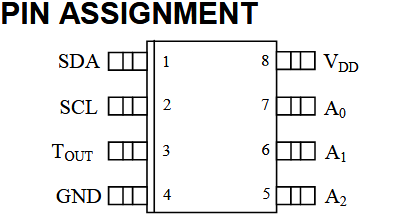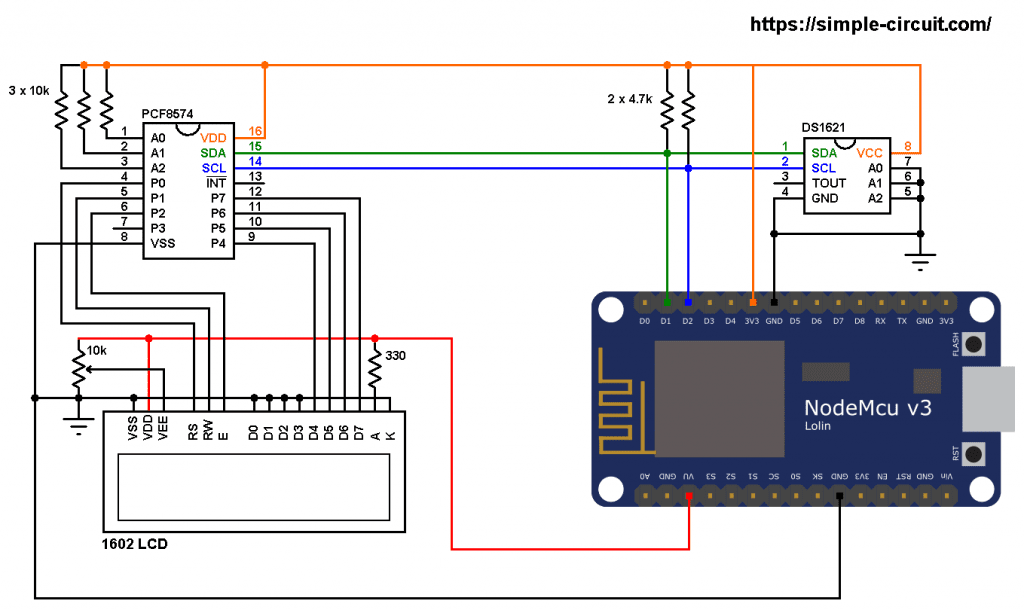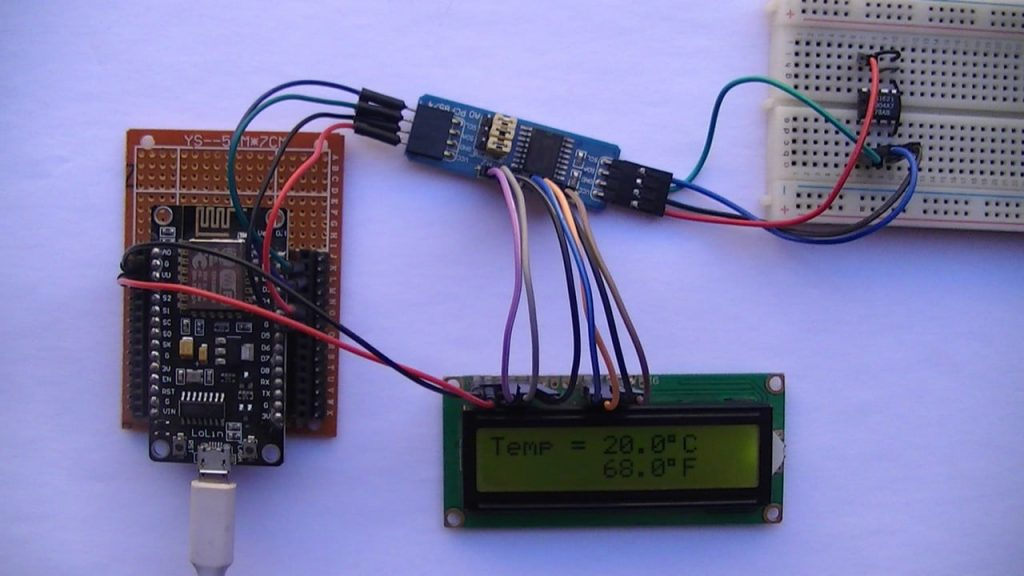# NodeMCU with I2C LCD and DS1621 Temperature Sensor

This project shows how to interface ESP8266 NodeMCU board (ESP-12E) with DS1621 digital temperature sensor where temperature values (in degrees Celsius and degrees Fahrenheit) are printed on 1602 LCD screen.
The 1602 LCD used in this project is connected to PCF8574 I2C I/O expander which allows it to communicate with NodeMCU board via I2C bus, this minimizes number of pins required and also may simplify the circuit.
The I2C bus uses only two pins: SDA (serial data) and SCL (serial clock).
This interfacing should also work with DFRobot I2C LCD displays.

The DS1621 Digital Thermometer and Thermostat from Maxim Integrated provides 9-bit temperature readings, which indicate the temperature of the device. The thermal alarm output, TOUT, is active when the temperature of the device exceeds a user-defined temperature TH. The output remains active until the temperature drops below user defined temperature TL, allowing for any hysteresis necessary.

Benefits and features:

• Measures temperatures from – 55 °C to +125 °C in 0.5 °C increments. Fahrenheit equivalent is -67 °F to 257 °F in 0.9 °F increments.
• Temperature is read as a 9-Bit value (2-byte transfer).
• Converts temperature to digital word in less than 1 second.
• Thermostatic settings are user definable and nonvolatile.
• Power supply range (2.7V to 5.5V).
• Data is read from/written via a 2-wire serial interface (open drain I/O lines).
• Saves space.
• Temperature measurements require no external components.
• 8-pin DIP or SO package (208-mil) packages.The I2C LCD display and the DS1621 sensor can be connected to the same I2C bus where the NodeMCU board microcontroller can talk with both devices (one at a time).

Hardware Required:

• ESP8266 NodeMCU board (LoLin)
• DS1621 temperature sensor   —->   datasheet
• 1602 LCD screen
• PCF8574 I/O expander (or PCF8574A)   —->   PCF8574 datasheet
• 3 x 10k ohm resistor
• 2 x 4.7k ohm resistor
• 330 ohm resistor
• 10k ohm variable resistor or potentiometer
• micro USB cable (for programming and powering the circuit)
• Jumper wires

NodeMCU with I2C LCD and DS1621 sensor circuit:
Project circuit diagram is shown below.The PCF8574 I/O expander is powered with 3.3V that comes from the NodeMCU board (PCF8574 VDD pin is connected to NodeMCU 3V3 pin and VSS is connected to GND).
SDA and SCL pins of the PCF8574 are respectively connected to NodeMCU pins D1 and D2.

A0, A1 and A2 pins of the PCF8574 are address select pins, they decide the I2C address of the chip. In this example each pin is connected to VDD (3.3V) through a 10k ohm resistor (the 10k resistor is optional, each pin can be connected directly to VDD).
The I2C address of the PCF8574 is: 0x20 | A2 A1 A0
In the circuit above the three pins A2, A1 and A0 are connected to VDD ( each one through 10k resistor). That means the I2C address is equal to 0x20 | 7 = 0x27.

If the PCF8574A is used instead of the PCF8574 then the I2C address is: 0x38 | 7 = 0x3F.

LCD data pins are connected to the PCF8574 where: RS, RW, E, D4, D5, D6 and D7 are respectively connected to P0, P1, P2, P4, P5, P6 and P7.
The 1602 LCD is powered with 5V from the NodeMCU board where its VDD pin is connected to NodeMCU pin VU and VSS is connected to GND.
The 1602 LCD operating voltage range is 4.5V – 5.5V and it may not work with 3.3V. The good thing is it accepts 3.3V on its I/O pins.

Other LCD pins are connected as follows:
VSS, RW, D0, D1, D2, D3 and K are connected to NodeMCU GND pin,
VEE to the 10k ohm variable resistor (or potentiometer) output,
A is connected to NodeMCU VU pin (5V) through 330 ohm resistor.

VEE pin is used to control the contrast of the LCD. A (anode) and K (cathode) are backlight LED pins.

The DS1621 is powered with 3.3V from the NodeMCU board, SDA and SCL pins are respectively connected to NodeMCU pins D1 and D2 (PCF8574 and DS1621 are connected to the same I2C bus).
Pins: A0, A1 and A2 are also address select pins, they are connected to GND, therefore DS1621 salve address is 0x48.

NodeMCU with I2C LCD and DS1621 sensor code:
To be able to compile project code with no error, a library for the I2C LCD display is required, download link is below:

Hints:
The I2C LCD library is initialized with address of 0x27, 2 rows and 16 columns:

Since the address pins of the DS1621 (A2, A1 and A0) are connected to NodeMCU GND, its I2C slave address is 0x48, it’s defined in the code as shown below:

A function named get_temperature() in the Arduino code is used to read raw temperature values from the DS1621 sensor and convert it to tenths degrees Celsius (output value of “135” equals 13.5 °Celsius).
This functions returns a signed integer number (2 bytes).

The temperature in tenths degrees Fahrenheit =  (tenth °Celsius) x 9/5 +320 (because: °F = °Cx9/5 + 32).

To get the actual value of each quantity we’ve to divide it by 10. The line below shows an example for temperature in °C:

We get the first 2 digits by dividing the tenths value by 10, and the tenths number (number after the decimal point) of the actual temperature value is equal to the reminder of that division (tenths value % 10).

The resolution of this thermometer is 0.5°C.

Full Arduino code:Related Project:

## One comment

1.Mohd Yameen says:

Dear can you make a project with LoRa and PIC CCS compiler please.?

This site uses Akismet to reduce spam. Learn how your comment data is processed.